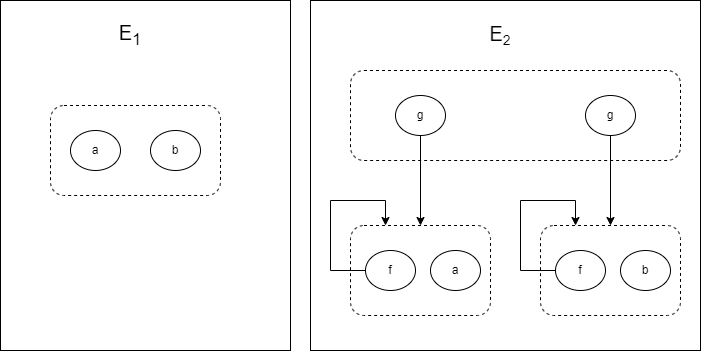8.5

## Limitations of E-Graph Intersection

This post took me a couple tries to get right! My original post claimed that I had a correct implementation of egraph intersection (which I now know is impossible).

### 1 E-Graphs

E-Graphs are a data structure for representing equalities between terms efficiently. This post assumes you know what an e-graph is, so here’s a great post about them.

### 2 E-Graphs and Proofs

E-Graph implementations (including egg) typically provide a way to generate a proof certificate for why two terms are equal. For example, the e-graph may know a + b is equal to b + a using an identity x + y = y + x. The proof would say it used the identity to infer the specific terms a + b and b + a are equal. Each particular use of the rule results in an equality like a + b = b + a. You can think of a proof certificate as a set of equalities on specific terms E = \{ T_1 = T_2, T_3 = T_4, ... \}.

E-Graphs compute the congruence closure over terms:

Thm1: If you insert a set of equalities E into the e-graph, then it will say two terms T_i and T_j are equal iff there is a valid proof between them (potentially using congruence).

We can also do the reverse: given an e-graph, we can extract back out the set of equalities that generate the e-graph. There are multiple choices for this set of equalities, but the simplest choice is the terms originally inserted into the e-graph either directly or by axiom instantiations.

### 3 E-Graph Union

Let’s write the union of two e-graph E_1 \cup E_2 = E_3. Intuitively, E_3 should represent terms who are equal due to a combination of facts from E_1 and E_2. More formally, any two terms T_1 and T_2 should be equal in E_3 iff there is a valid proof that they are equal involving only facts from E_1 and E_2.

There’s a simple algorithm to union e-graphs, and it relies on the set of equations that originally generated the e-graph \{ T_1 = T_2, T_3 = T_4, ... \}. First, make a new e-graph E_3. Now assert every equality T_i = T_j from E_1 in E_3. Similarly, assert every equality T_k = T_m from E_2 in E_3. Do this by adding the two terms to E_3 and unioning them in the e-graph.

Here’s some rust code that does it for egg (though it’s not merged into main yet):

By our Thm1, this new e-graph will tell you when two terms are equal because of a combination of facts from each e-graph.

### 4 E-Graph Intersection

Ideally, we would want the intersection of two e-graphs to represent all equalities that are true in both e-graphs. Unfortunately, there’s no way to guarantee this with a finite e-graph, as shown in this paper. Take for example E_1 = \{ a = b \} and E_2 = \{f(a) = a, f(b) = b, g(a) = g(b)\}. The e-graphs look like this:The intersection of E_1 and E_2 is the infinite set E_3 = \{ g(f^n(a)) = g(f^n(b)) | n \geq 1\}. However, this isn’t finitely representable in the e-graph because it can no longer rely on the cycle f(a) = a. If we tried, we would end up with terms f(f(a)) = g(f(b)), g(f(f(a))) = g(f(f(b))), and so on in the egraph.

Since computing the intersection is infeasible, the e-graph intersection paper mentioned above calculates it for a set of important terms I. The intersection can be calculated simply by checking every possible pair of equalities between terms in I. In fact, the optimal algorithm for computing the intersection is still quadratic in the size of I

Instead of doing all pairs in a set I, you can perserve a set of particular equalities between terms instead. A good choice for equalities to preserve are the original terms that generated the egraphs E_1 and E_2. That way, proofs involving only equalities shared by both egraphs are preserved, which is a weak but potentially useful property. Here’s some code that performs this version:

Note that in the code above, we need to maintain congruence closure in the second e-graph by calling the rebuild function. It’s crucial so that we can query it for which equalities are true.

### 5 Runtime Analysis

If we assume that all the equalities in E_1 and E_2 are bounded in size by some constant, then the overall runtime of these union and intersection algorithms is O(n \log{n}), the run time of maintaining congruence closure for n equalities (see this paper). We can, however, get a faster implementation by carefully tracking the new identities of sub-terms in our target e-graph. This saves us the additional cost of copying the same sub-terms over to the new e-graph each time.